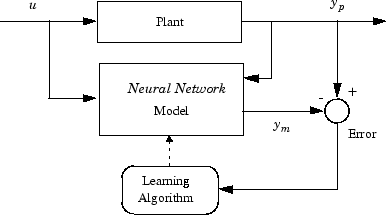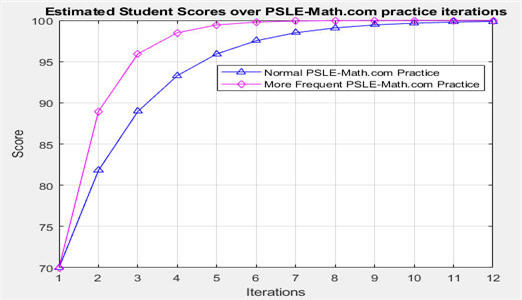# PSLE-Math.com

PSLE-Math.com is an online Math Tutor and provides Math Tuition. The platform generates PSLE math, P6 math, P5 math and P4 math questions by Machine Learning and Artificial Intelligence technologies. Students can practice the same type of questions repetitively and unlimitedly. It can be scientificaly proven by using Neural Network theory, after sufficient training of the same type, the student's brain will convergce to 100% accuracy.

Meanwhile, through PSLE Math practice, students can improve their mental sum capability significantly.